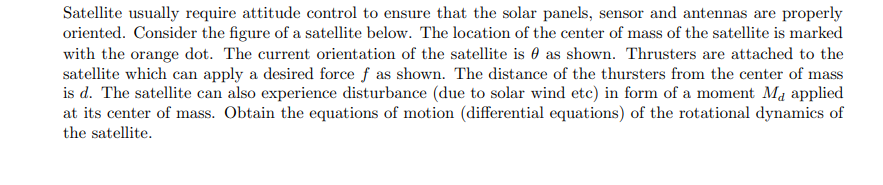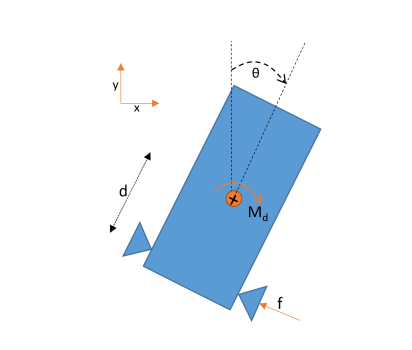# (Solved): Satellite usually require attitude control to ensure that the solar panels, sensor and antennas a ...Satellite usually require attitude control to ensure that the solar panels, sensor and antennas are properly oriented. Consider the figure of a satellite below. The location of the center of mass of the satellite is marked with the orange dot. The current orientation of the satellite is $$\theta$$ as shown. Thrusters are attached to the satellite which can apply a desired force $$f$$ as shown. The distance of the thursters from the center of mass is $$d$$. The satellite can also experience disturbance (due to solar wind etc) in form of a moment $$M_{d}$$ applied at its center of mass. Obtain the equations of motion (differential equations) of the rotational dynamics of the satellite.

We have an Answer from Expert